# 19.2: Acidity of Carboxylic Acids

$$\newcommand{\vecs}{\overset { \rightharpoonup} {\mathbf{#1}} }$$ $$\newcommand{\vecd}{\overset{-\!-\!\rightharpoonup}{\vphantom{a}\smash {#1}}}$$$$\newcommand{\id}{\mathrm{id}}$$ $$\newcommand{\Span}{\mathrm{span}}$$ $$\newcommand{\kernel}{\mathrm{null}\,}$$ $$\newcommand{\range}{\mathrm{range}\,}$$ $$\newcommand{\RealPart}{\mathrm{Re}}$$ $$\newcommand{\ImaginaryPart}{\mathrm{Im}}$$ $$\newcommand{\Argument}{\mathrm{Arg}}$$ $$\newcommand{\norm}{\| #1 \|}$$ $$\newcommand{\inner}{\langle #1, #2 \rangle}$$ $$\newcommand{\Span}{\mathrm{span}}$$ $$\newcommand{\id}{\mathrm{id}}$$ $$\newcommand{\Span}{\mathrm{span}}$$ $$\newcommand{\kernel}{\mathrm{null}\,}$$ $$\newcommand{\range}{\mathrm{range}\,}$$ $$\newcommand{\RealPart}{\mathrm{Re}}$$ $$\newcommand{\ImaginaryPart}{\mathrm{Im}}$$ $$\newcommand{\Argument}{\mathrm{Arg}}$$ $$\newcommand{\norm}{\| #1 \|}$$ $$\newcommand{\inner}{\langle #1, #2 \rangle}$$ $$\newcommand{\Span}{\mathrm{span}}$$$$\newcommand{\AA}{\unicode[.8,0]{x212B}}$$

## Comparing the strengths of weak acidsThe strengths of weak acids are measured on the pKa scale. The smaller the number on this scale, the stronger the acid is. Three of the compounds we shall be looking at, together with their pKa values are:Remember - the smaller the pKa, the stronger the acid. Comparing the other two to ethanoic acid, we see that phenol is very much weaker with a pKa of 10.00, and ethanol is so weak with a pKa of about 16 that it hardly counts as acidic at all! The pKa of ethanol is about 17, while the pKa of acetic acid is about 5: this is a 1012-fold difference in the two acidity constants. In both compounds, the acidic proton is bonded to an oxygen atom. How can they be so different in terms of acidity? We begin by considering the conjugate bases.

In both species, the negative charge on the conjugate base is held by an oxygen, so periodic trends cannot be invoked. For acetic acid, however, there is a key difference: a resonance contributor can be drawn in which the negative charge is localized on the second oxygen of the group. The two resonance forms for the conjugate base are equal in energy. What this means is that the negative charge on the acetate ion is not located on one oxygen or the other: rather it is shared between the two. Chemists use the term ‘delocalization of charge’ to describe this situation. In the ethoxide ion, by contrast, the negative charge is ‘locked’ on the single oxygen – it has nowhere else to go.

Recall the fundamental idea that electrostatic charges, whether positive or negative, are more stable when they are ‘spread out’ than when they are confined to one atom. Here, a charge is being ‘spread out’ (in other words, delocalized) by resonance, rather than simply by the size of the atom involved.

The delocalization of charge by resonance has a very powerful effect on the reactivity of organic molecules, enough to account for the difference of over 12 pKa units between ethanol and acetic acid. The acetate ion is that much more stable than the ethoxide ion, all due to the effects of resonance delocalization.

## Resonance Effects on the Acidity of Carboxylic Acids

Why should the presence of a carbonyl group adjacent to a hydroxyl group have such a profound effect on the acidity of the hydroxyl proton? To answer this question we must return to the nature of acid-base equilibria and the definition of pKa , illustrated by the general equations given below.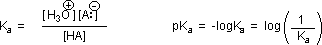We know that an equilibrium favors the thermodynamically more stable side, and that the magnitude of the equilibrium constant reflects the energy difference between the components of each side. In an acid base equilibrium the equilibrium always favors the weaker acid and base (these are the more stable components). Water is the standard base used for pKa measurements; consequently, anything that stabilizes the conjugate base (A:(–)) of an acid will necessarily make that acid (H–A) stronger and shift the equilibrium to the right. Both the carboxyl group and the carboxylate anion are stabilized by resonance, but the stabilization of the anion is much greater than that of the neutral function, as shown in the following diagram. In the carboxylate anion the two contributing structures have equal weight in the hybrid, and the C–O bonds are of equal length (between a double and a single bond). This stabilization leads to a markedly increased acidity, as illustrated by the energy diagram displayed by clicking the "Toggle Display" button.## Inductive Effects on Relative Acidity

The resonance effect described here is undoubtedly the major contributor to the exceptional acidity of carboxylic acids. However, inductive effects also play a role. For example, alcohols have pKa's of 16 or greater but their acidity is increased by electron withdrawing substituents on the alkyl group. The following diagram illustrates this factor for several simple inorganic and organic compounds (row #1), and shows how inductive electron withdrawal may also increase the acidity of carboxylic acids (rows #2 & 3). The acidic hydrogen is colored red in all examples. Water is less acidic than hydrogen peroxide because hydrogen is less electronegative than oxygen, and the covalent bond joining these atoms is polarized in the manner shown. Alcohols are slightly less acidic than water, due to the poor electronegativity of carbon, but chloral hydrate, Cl3CCH(OH)2, and 2,2,2,-trifluoroethanol are significantly more acidic than water, due to inductive electron withdrawal by the electronegative halogens (and the second oxygen in chloral hydrate). In the case of carboxylic acids, if the electrophilic character of the carbonyl carbon is decreased the acidity of the carboxylic acid will also decrease. Similarly, an increase in its electrophilicity will increase the acidity of the acid. Acetic acid is ten times weaker an acid than formic acid (first two entries in the second row), confirming the electron donating character of an alkyl group relative to hydrogen, as noted earlier in a discussion of carbocation stability. Electronegative substituents increase acidity by inductive electron withdrawal. As expected, the higher the electronegativity of the substituent the greater the increase in acidity (F > Cl > Br > I), and the closer the substituent is to the carboxyl group the greater is its effect (isomers in the 3rd row). Substituents also influence the acidity of benzoic acid derivatives, but resonance effects compete with inductive effects. The methoxy group is electron donating and the nitro group is electron withdrawing (last three entries in the table of pKa values).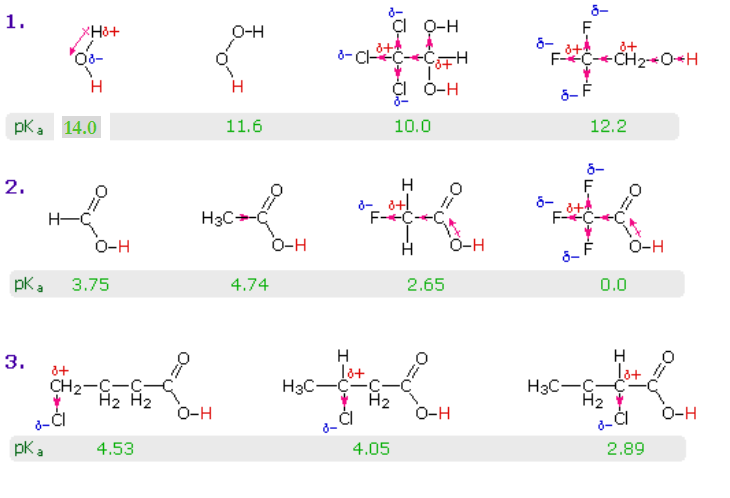A closer look at the effects of electron-withdrawing and electron-donating groups on the stability of the conjugate bases can be seen in the pKa values of benzoic acid as shown in the table below. The conjugate base of benzoic acid is stabilized by electron-withdrawing groups. This makes the acid more acidicElectron-withdrawing groups deactivate the benzene ring to electrophilic reactions and make benzoic acids more acidic.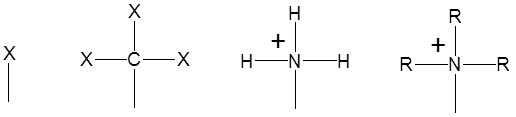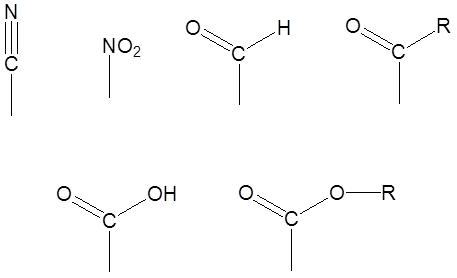The conjugate base of benzoic acid is destabilized by electron-donating groups. This makes the acid less acidicElectron-donating groups activate the benzene ring to electrophilic reactions and make benzoic acids less acidic.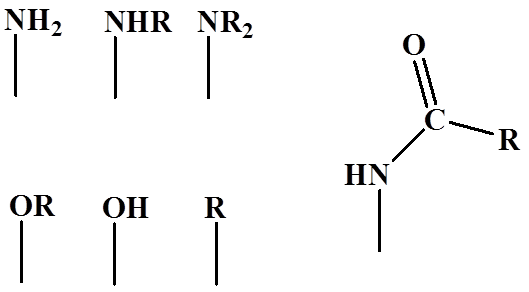Exercise

2. Draw the bond-line structures and arrange the following compounds in order of increasing acidity: 4-nitrobenzoic acid; 4-(methylamino)benzoic acid; p-chlorobenzoic acid; 4-(dimethylamino)benzoic acid.